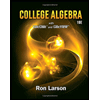Question
Assume that random guesses are made for seven multiple choice questions on an SAT​ test, so that there are n=7 trials, each with probability of success​ (correct) given by p=0.25
Find the probability that the number x of correct answers is fewer than
4.

Expert Solution

### Want to see the full answer?

Check out a sample Q&A hereStudents who’ve seen this question also like:College Algebra
10th Edition
ISBN: 9781337282291
Author: Ron Larson
Publisher: Cengage Learning